# Exponents

##By Khan Academy

Taking an exponent is basically the act of repeated multiplication. You know how to multiply, right? If so, understanding exponents is completely within your grasp!# Exponents: Multiplying Variables with Rational Exponents – Basic Ex 2

##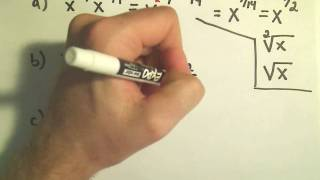By PatrickJMT

Exponents: Multiplying Variables with Rational Exponents – Basic Ex 2# DEPRECATED Simplifying expressions with exponents

##By Khan Academy

Exponent Properties 3# DEPRECATED Simplifying expressions with exponents

##By Khan Academy

Exponent Properties 4# DEPRECATED Simplifying expressions with exponents

##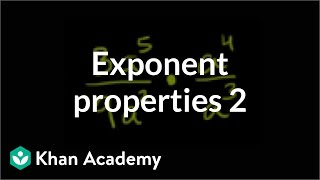By Khan Academy

Exponent Properties 2# ACT Prep - Exponents

##By Dr.Steeve Warner

ACT Prep - Exponents# Algebra 2 - Rational Exponents

##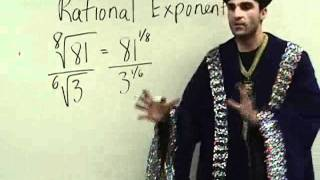By Yaymath

Join the mathemagician's adventure into simplifying expressions with different exponents. Fraction exponents, negative exponents, and roots of varying powers. YAY MATH!# More on Rational Exponents

##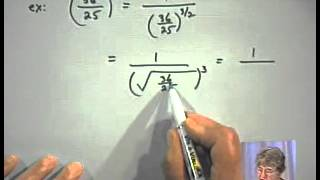By Learners TV

More on Rational Exponents# Algebra 2: Properties of Exponents

##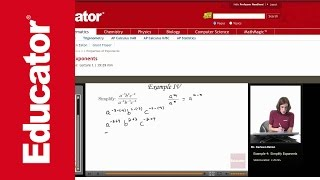By Educator

Algebra 2: Properties of Exponents# Exponents: Multiplying Variables with Rational Exponents – Basic Ex 1

##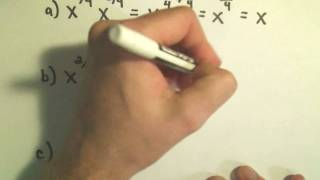By PatrickJMT

Exponents: Multiplying Variables with Rational Exponents – Basic Ex 1# Simplify Rational Exponents

##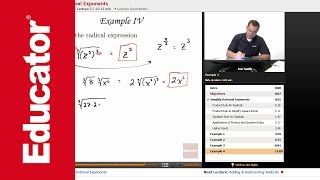By Educator

Simplify Rational Exponents# DEPRECATED Simplifying expressions with exponents

##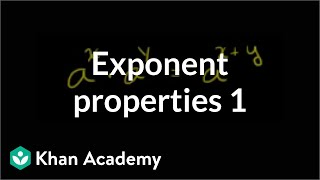By Khan Academy

When multiplying numbers with common base, add exponents# Class VIII-Math-Exponents and Powers

##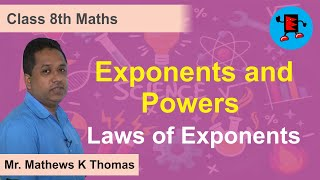By Extraminds

Class VIII-Math-Exponents and Powers# More rational exponents and exponent laws | Algebra I | Khan Academy

##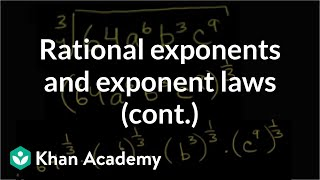By Khan Academy

Khan Academy presents More Rational Exponents and Exponent Laws, an educational video resource on math.# "Rational Exponents" | Algebra 1 with Educator.com

##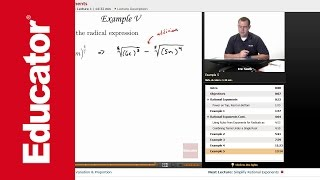By Educator

"Rational Exponents" | Algebra 1 with Educator.com# Definition, Evaluation of numbers with Rational Exponents

##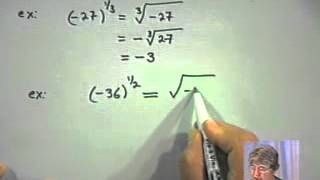By Learners TV

Definition, Evaluation of numbers with Rational Exponents##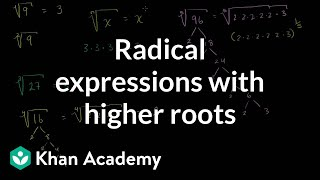By Khan Academy

Sal explains the relationship between radical notation and unit-fraction exponents, including a discussion of what the principal square root is. Then he shows how to simplify the 6th root of (64x^8) using the properties of exponents.# Properties of Rational Exponents

##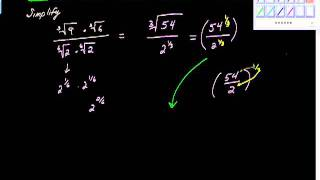By charlie Lindelof

YouTube presents Properties of Rational Exponents, an educational video resource on mathematics.# Simplifying a radical expression using rational exponents

##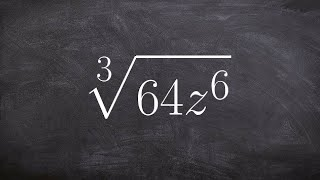By Brain McLogan

Learn how to simplify rational powers using the power and the product rules. There are some laws of exponents which might come handy when simplifying expressions with exponents. Some of the laws include the product law which states that the product of numbers/expressions having the same base is equivalent to taking one of the base with the sum of the original exponents as the new exponent. The power law says that when an expression with an exponent is raised to another exponent that it is equivalent to the expression with the product of the original exponents as the new exponent. Knowledge of these exponent laws will help you in simplifying power expressions using the power rule and the quotient rule.# Exponent rules part 1 | Exponents, radicals, and scientific notation | Pre-Algebra | Khan Academy

##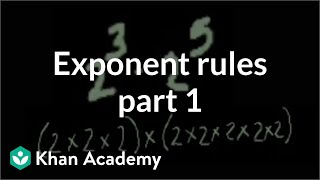By Khan Academy

Introduction to exponent rules